# Stirling Numbers of the Second Kind

The Stirling numbers of the second kind, or Stirling partition numbers, describe the number of ways a set with n elements can be partitioned into k disjoint, non-empty subsets. Common notations are S(n, k),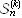, and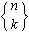, where the first is by far the easiest to type.

For example, the set {A, B, C} can be partitioned into one subset (that is, not partitioned at all) in the following way, which means S(3, 1) = 1:

• {A, B, C}

into two subsets in the following ways (making S(3, 2) = 3):

• {A, B} {C}
• {A, C} {B}
• {A} {B, C}

and into three subsets in the following way (S(3, 3) = 1):

• {A} {B} {C}

Here are some diagrams representing the different ways the sets can be partitioned: a line connects elements in the same subset, and a point represents a singleton subset.

 S(3, 1):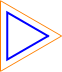S(3, 2):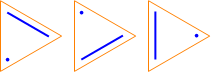S(3, 3):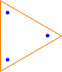S(4, 1):S(4, 2):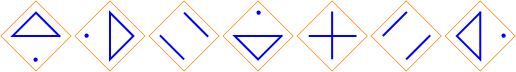S(4, 3):S(4, 4):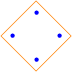S(5, 1):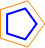S(5, 2):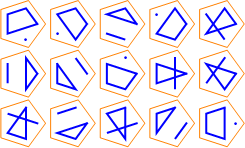S(5, 3):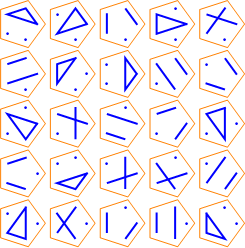S(5, 4):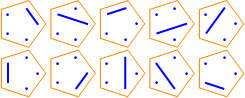S(5, 5):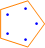You’ll notice that S(n, 1) is always 1: there’s only one way to partition a set into one subset, which is to leave it alone.

You’ll also notice that S(n, n) is also always 1: there’s only one way to partition a set of length n into n subsets, each of length 1.

The Stirling numbers of the second kind also count numbers of ways to place nonattacking rooks on a triangular checkerboard. (Compare with the S(4, k) diagrams.)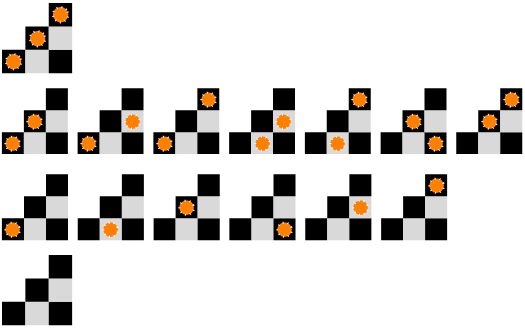(Does the game of checkers use rooks?)

The numbers can be computed recursively using the formula:

S(n, k) = S(n−1, k−1) + S(n−1, k)

The idea is that you can build up S(n, k) by:

• Taking each of the S(n−1, k−1) partitions and adding singleton {n}
• Taking each of the S(n−1, k) partitions and appending element n to each of the k subsets in turn

For example, the following shows how to compute S(4, 3) by appending singleton {e}—represented by the orange dot at the bottom of each square—to each of the three S(3, 2) partitions, plus appending element e to each of the three S(3, 3) subsets: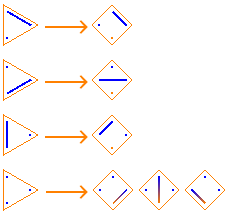The sums of the Stirling numbers of the second kind,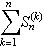are called the Bell numbers.

About the checkerboard interpretation, see M. B. Wells, Elements of Combinatorial Computing, Oxford: Pergamon Press, 1971 p. 185.

Designed and rendered using Mathematica 3.0 and 7.0.